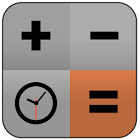Time Calculator

All Android applications categories

All Android games categories# Time Calculator

by: 879 8

8 Users
rating

## Screenshots

Description

Professional time calculator.

It contains a simple general calculator and is a time calculator that can add, subtract, multiply, divide, and convert time units.

Time Calculator is essential for professional occupations related to time measurement!

You do not have to convert time units (years, days, hours, minutes, seconds) and look up another regular calculator again.

function :

Calculator that can calculate year, day, hour, minute, second
Combination of time units
Convert to other time units (year, day, hour, minute, second)
Record the formula of the calculation and reuse it by selecting it from the record at any time
Can also be used as a general calculator

※ For those who use this calculator for the first time, you need to know a few things:

Enter the method for entering the time in the following order.

To enter 7 hour 6 minute 3 seconds, type:

1. Enter the number 
2. Enter the hour
3. Enter the number 
4. Enter the minute
5. Enter number 
7. Enter the seconds

If you press [Convert] button, you can convert to a specific unit and select specific conversion unit value and add.

Example) 7 hours 6 minutes 3 seconds -> [convert] -> 25,563 seconds

A variety of complex formulas are also available.

For example:

(1 year + 1 day) x 8% = 29 days 6 hours 43 minutes 12 seconds (conversion can be verified by other specific units)
2 days x 2 = 4 days
2 days - 30 minutes = 23:30 on the 1st

Decimal point calculation example) 7.3 hours + 8.9 hours = 16 hours 12 minutes

Percentage calculation example) x 50% for 50 minutes = 25 minutes

Basic calculation example) 150 - 5 x 5 x 4 + 13.6 = 2913.6

from 879 reviews

"Great"

8